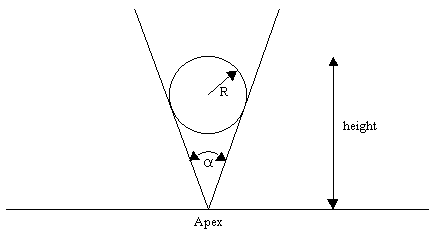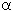Not an official ACM page
[Problem A | 1995 East-Central problem set | My ACM problem archive | my home page]

## 1995 East-Central Regionals ACM International Collegiate programming Contest

### Practice Problem 2 - The Marble

Consider a right circular cone standing upright on its apex. A marble is to be dropped into the cone, and we are to find the height of the top of the marble after it comes to rest in the cone.The angle at the apex of the cone,, and the radius of the marble, R, will be given within the following limits:

45.000° <=<= 90.000°

0.001 <= R <= 1.000

In this problem, you'll receive a series of data sets. Each data set contains two real values on a single line, the angle, given in degrees, followed by the radius, R. For each set of data values, give the resulting height as the output. The units of height are whatever the units used for R are, and are unspecified.

### Input

The input consists of a series of data sets. Each data set has two real values on a line, the value for, in degrees, followed by the value for R. The default real/float input format should be used.

Both input values will be zero to indicate the end of data.

### Output

A line with a single real value is to be emitted for each input data set encountered. This value to be reported is the height of the top of the marble. The default real/float output format should be used. Answers must be within 0.1% of the correct answer.

### Example

 Input File Output File ```90.000 1.000 0.000 0.000``` `2.414`